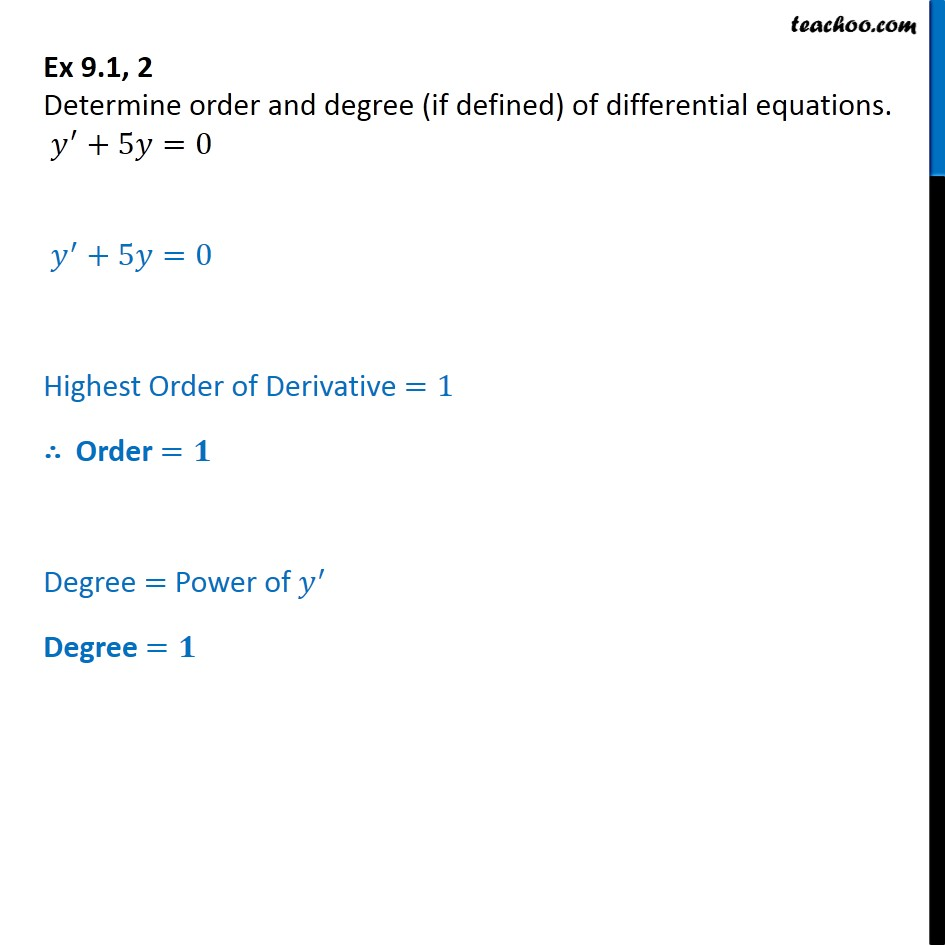Ex 9.1

Chapter 9 Class 12 Differential Equations
Serial order wiseLearn in your speed, with individual attention - Teachoo Maths 1-on-1 Class

### Transcript

Ex 9.1, 2 - Chapter 9 Class 12 Differential Equations - NCERT Determine order and degree (if defined) of differential equations. y′ + 5𝑦 = 0 Highest Order of Derivative =1 ∴ Order = 1 Degree = Power of y' Degree = 1